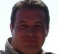## How to estimate the SNRStarted by 7 years ago10 replieslatest reply 7 years ago1846 views

Hi

I would like to know can anyone suggest me techniques to estimate the #SNR for a given noisy signal. I do not know the amplitude of my signal but I do know that noise is Gaussian. I have tried to do estimation in frequency domain where I simply take the FFT of the signal and find the dominant peak and find the power associated with that as the signal power and noise power as the power in all other bins. However this technique seems to work well when I have sinusoidal signal and fails easily when I just have a noisy signal and do not know the nature of the signal since I do not know what peaks in FFT corresponds to my signal and how many of them are for the signal.
[ - ]In general, the correct answer is "I'm sorry, that can't be done."

You need to know enough about the signal to at least calculate it's power.  If you can't, then you don't have enough information to calculate SNR.

Now, if you have a signal of some known form, then it may be possible -- but if you're just trying to find the ratio of any old signal to any old noise, then you're probably out of luck.

[ - ]Yes the signal is the noisy BPSK signal so noise at the top of -1 and 1 however we do not know about other factors that might be multiplied by the signal meaning that if you assume received signal to be r and transmitted signal to be c then usually one might write down r=c+n however now I am dealing with the case of r=Ac+n where A might be the gain. I tried to estimate the A using the least square estimation but we do not have c. Therefore signal power is A^2.

[ - ]Hi Chess,

From what you wrote, you have r = Ac+n and you want to estimate SNR = (Power of c)/(Power of n), or perhaps SNR = (Power of Ac)/(Power of n). But A, c, and n are unknown! Computing an SNR looks like an impossible task to me. Tell us Chess, are your signals "real-world" (captured with an A/D converter) signals or are they signals you generated with some signal processing software?

[ - ]Hi Rick

It is a "real-world" signal.

[ - ]Then -- assuming you know the pulse shape and the bit rate -- you know a hell of a lot about your signal.

Assuming that you can get frequency and bit-clock lock on the signal, that you use a matched filter to receive bits, and that your matched filtering is accurate, you have enough information to estimate A and n.  It's even easier if you're getting accurate bit reception.

I've done this.  It's been decades, so I can't give you the details -- just pull out your detection and estimation textbook, and work out the expected probability distribution of the output of your matched filter at bit-sample time.  Then find metrics on that probability distribution that give you A and n (as I said, I can't remember the details).

If the SNR is good enough for accurate bit reception, and if you want the SNR over the bandwidth of the matched filter, then A is just the average absolute value of the matched filter, and n is the variance of that matched filter.  If you want the SNR over some wider bandwidth and you can assume that the noise is white, you can just scale that variance.

As the bit error rate gets worse the above calculation gets less accurate, but IIRC the absolute value of the matched filter, and it's variance, are still pretty good metrics -- you just need to correct them somewhat to get the right answer.

[ - ]Hi Chess,

I believe SNR measurements for a system is always done with a single tone input and the procedure mentioned by you works fine for a single tone.

Please correct me if I am wrong. Also, it is always recommended to use a windowing for the FFT of a noisy signal. In which case, the signal on bin also gets smeared into adjacent bins. (Number of bins depending on the window used). With windowing you need to consider the power in those bins too.

Regards,

Krishna

[ - ]SAs Tim Wescott pointed out, unless you know something apriori from the signal, there's no way you can do this. What you mention about exploring the frequency spectrum in quiet moments would give you an estimation on the signal to noise plus interference ratio (SINR).

Once I did an SNR estimator based on the apriori knowledge of the signal. It was for OFDM signals, particularly WiFi and WiMax and on that side, look for Error Vector Magnitude. This works quite well in practice but assumes the noise PDF to be symmetric. Hope this works for your case.

[ - ]If your fft method worked for single tone SNR then it can work for your band limited signal provided you target out-of-band noise.

Using fft method, you must subtract its artefact:

for single tone you must subtract 10*log(fft resolution/2)

for bandlimited signal remove 10*log(Nyquist/bw)

Kaz

[ - ]Hi Kaz

Can you explain about the artefact and what you mean by that. I know detrend the signal by subtracting its mean. But can you explain about Following :

for single tone you must subtract 10*log(fft resolution/2)

for bandlimited signal remove 10*log(Nyquist/bw)

what is resolution, do you mean delta_f (length of a bin). Nyquist do you mean Fs/2 and what do you mean by bw ? if you mean bandwidth of my signal it is not given but I can take FFT over any range so range of frequencies for two sided bandwidth is Fs.

[ - ]